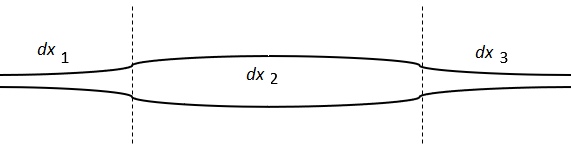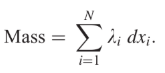# Line Integral (Path Integral): Simple Definition, Examples

Contents:

## What is a Line Integral (A Path Integral)?

A line integral (also called a path integral) is the integral of a function taken over a line, or curve.

The integrated function might be a vector field or a scalar field; The value of the line integral itself is the sum of the values of the field at all points on the curve, weighted by a scalar function. That weight function is commonly the arc length of the curve, or—if you’re integrating over a vector field—the scalar product with a vector differential in the curve.

It’s this weighting which sets a line integral apart from the integrals studied early on in calculus—simpler integrals defined on intervals.

## Example of a Line Integral

A simple example of a line integral is finding the mass of a wire if the wire’s density varies along its path.If you were to divide the wire into x segments of roughly equal density (as shown above), you could sum all of the segment’s densities to find the total density using the following mass function:Where:

• dxi = length of each segment
• λi = linear density of each segment.

However, if those line segments approach a length of zero, you could integrate to find a more accurate number for density.## Applications of Line Integrals in Physics

Many simple physical formulas can be written in terms of line integrals. For example, work is force times distance:
W = F · s.
If an object is moving through an electric or gravitational field, you can write it as:## The Line Integral in a Scalar Field

The animation below shows how a line integral over some scalar field f can be thought as the area under the curve C along a surface z = f(x,y), where z is described by the field.If C is a smooth, piecewise curve, and f is a scalar field such thatthenHere r: [a,b]→ C is an arbitrary bijective parameterization of the cure C such that—and this is key—r(a) and r(b) give the endpoints of C. a is also defined to be less than b.

## What are Path Independent Integrals?

A path independent integral is one where you can take different paths and get the same result. Let’s say you wanted to evaluate the following integral along a path between two points:That integral is path independent if the integral is equal to the same integral taking a different path with the same endpoints.In order to establish path independence, evaluate both integrals (using the multivariate chain rule). If they are equal, then the integral is path independent. If they aren’t equal, then there isn’t path independence.

For example, these two integrals are not path independent, because they give different results:## References

Fleisch, D. (2008). A student’s Guide to Maxwell’s Equations. Cambridge University Press.
Sharma, A. Text Book of Vector Calculus.